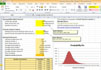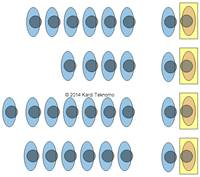M/M/s Queuing Optimization Spreadsheet is now available only at \$9.99

## M/M/1 Queuing System

Now we will go into the detail of the performance for a special simple queuing system where there is only single server and the arrival distribution of customers follows Poisson distribution and the distribution for service time follows Exponential distribution.M/M/1 queuing system means we have one queue per server. It does not mean that you cannot have multiple servers. A diagram above shows 4 servers with 4 queues. Therefore, each of these servers are computed using M/M/1 queues.

Use the M/M/1 queuing calculator below to experiment to solve queuing problem of a single server. For instance, what happen to the queuing performance if you can improve the service rate by 20%? You can also compare the performance of 4 servers with 4 queues (4*M/M/1) with the performance of 4 servers with 1 queue (M/M/4). Check the queuing calculator for M/M/s here .

M/M/1 Queuing system is also equivalent to M/G/1 queuing system with standard deviation of service rate. The formulation of measurement of effectiveness of M/M/1 queuing system are given below.

Input:

• Arrival rate (number of customers/unit time)• Service rate (number of customers/unit time)Output:

• U= Utilization factor = percentage of the time that all servers are busy,• P 0 = probability that there are no customers in the system,• P n = probability that there are n customers in the system,• W q = average time a customer spends in waiting line waiting for service,• W = average time a customer spends in the system (in waiting line and being served),• L q = average number of customer in waiting line for service,• L = average number of customer in the system (in waiting line and being served),Example:

Our observations of one cashier in a supermarket have shown that the arrival distribution of customers follows Poisson distribution with arrival rate ofcustomers/5 minutes. The distribution of service time follows Exponential distribution with average service time= 1.079 minutes per customer. Calculate the measurement of effectiveness of the queuing system.

Solution:

This problem is M/M/1 queuing system because we have only single server with Poisson arrival distribution and Exponential service time distribution. The arrival rate iscustomers/5 minutes = 0.15 customers/minute. Putting the same unit, we have average service time of= 1.079 minutes per customer which is equivalent tocustomers per minute.

Utilization factor = percentage of the time that all servers are busy,P 0 = probability that there are no customers in the system,Average time a customer spends in waiting line waiting for service,minutes.

Average time a customer spends in the system (in waiting line and being served),minutes.

L q = average number of customer in waiting line for service,customers.

L = average number of customer in the system (in waiting line and being served),customers.

P n = probability that there are n customers in the system,Preferable reference for this tutorial is

Teknomo, Kardi. (2014) Queuing Theory Tutorial
http://people.revoledu.com/kardi/tutorial/Queuing/